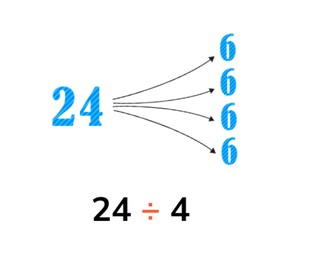Solving division with the division tables

# Solving division with the division tables

The students learn to solve division problems with the division tables

No account needed.8,000 schools use Gynzy92,000 teachers use Gynzy1,600,000 students use Gynzy

## General

The students learn to calculate with the division tables 1 to 10.

## Standards

CCSS.Math.Content.3.OA.B.5/6

## Learning objective

Students will be able to solve division problems with the division tables of 1 to 10.

## Introduction

On the interactive whiteboard there are images from which you can make multiplication problems, such as three hands (3 × 5), four bikes (4 × 2) and strings of beads (5 × 7). Have the students come up with the matching problems and then solve them.

## Instruction

You explain what the numbers in a division problem mean. The first number in the problem is how much you have of something. The number after the division sign is how many times the amount is divided. The outcome is how much there is in each group. You first show this in a visual division problem. You can drag the fish from the pond to the fishbowls to show how you divide up the fish. You can calculate the visual division problems on the interactive whiteboard together with the students. For the next images you and the students are going to think together to determine which division problem matches the picture and for the following images you determine the division problem and then solve it together with the students. Next you explain how you can calculate an abstract division problem. You show how you divide the number into groups and then practice this together with the students. You also explain that a division problem is a backwards multiplication problem. You also discuss this through different problems. At the end of the instruction you discuss a story problem that is a division problem. You walk through the steps for which you first determine what type of problem it is, then what numbers you need, what the problem is that you are going to solve and finally what the answer is.

To check whether students can solve division problems, you complete the exercise in which the students must drag the outcomes to the problems and you can also ask the following questions:
- How can you solve a division problem?
- What does the first number of the problem mean?
- What number tells how many groups you have?

## Quiz

The students test their understanding of solving division problems with the division tables through ten exercises. Some of these exercises are abstract, others have visual support, and others are story problems.

## Closing

You recall the goal and the importance of it. Next there are division problems and multiplication problems on the interactive whiteboard. The students determine which multiplication problems match the division problems. You can drag the multiplication problems after the matching division problems. You may also then have the students solve the problems.

## Teaching tips

When the students have difficulty with solving division problems, you can give them blocks or chips. They can use these blocks or chips to make equal groups.

## Instruction materials

Blocks or chips

### The online teaching platform for interactive whiteboards and displays in schools

• Save time building lessons

• Manage the classroom more efficiently

• Increase student engagement